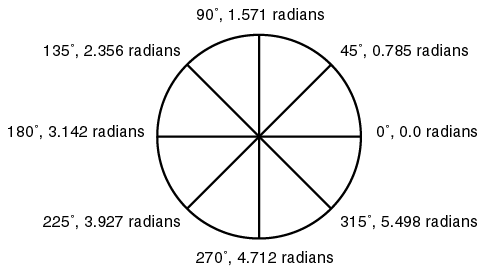## Complex Attributes

There are six available attributes for any complex number:

• real
• imaginary
• magnitude
• sum_of_squares
• degrees

Below is a diagram showing the complex number [4, 3]. The attribute values for real, imaginary, magnitude and degrees are labeled for that point.

The sum_of_squares attribute is simply the magnitude attribute without taking the square root. For the point [4, 3] shown in the diagram the values of magnitude and sum_of_squares are as follows:

attribute formula value
magnitude sqrt(4 * 4 + 3 * 3) 5
sum_of_squares 4 * 4 + 3 * 3 25

The reason for having both sum_of_squares and magnitude is that since the magnitude calculation involves taking a square root it is slower than the sum_of_squares calculation. It is often possible to create an equivalent (but more quickly generated) fractal image by using sum_of_squares instead of magnitude

The relationship between degrees and radians is based upon this equation:

``` 360 degrees = 6.28 radians ```

Here is a diagram showing a number of angles in degrees and their equivalent values in radians:The formulas for converting between degrees and radians are as follows:

``` degrees = 57.2958 * radians ```

and

``` radians = 0.017453 * degrees ```

The reason for having both radians and degrees is that the trigonometric functions (sin, cos, etc.) all expect the values supplied to them to be measured in radians.

Finally, nearly all the attributes have a long and short form

attribute short form examples
real real real(z), real([1, 2])
imaginary imag imaginary(z), imag([2, 3])
magnitude mag magnitude(z), mag([3, 4])
sum_of_squares ssq sum_of_squares(z), ssq([4, 5])
degrees deg degrees(z), deg([5, 6])